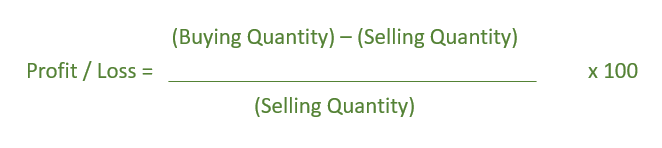# Program to find the profit or loss when CP of N items is equal to SP of M items

• Last Updated : 13 Apr, 2021

Givenanddenoting that the Cost Price of N articles is equal to the Selling Price of M articles. The task is to determine the profit or Loss percentage.

Examples:

Want to learn from the best curated videos and practice problems, check out the C++ Foundation Course for Basic to Advanced C++ and C++ STL Course for the language and STL. To complete your preparation from learning a language to DS Algo and many more, please refer Complete Interview Preparation Course.
```Input:  N = 8, M = 9
Output: Loss = -11.11%

Input:  N = 8, M = 5
Output: Profit = 60%```

Formula:-Below is the implementation of the above approach:

## C++

 `// C++ implementation of above formula``#include ``using` `namespace` `std;` `// Function to calculate``// Profit or loss``void` `profitLoss(``int` `N, ``int` `M)``{``    ``if` `(N == M)``        ``cout << ``"No Profit nor Loss"``;``    ``else` `{` `        ``float` `result = 0.0;` `        ``result = ``float``(``abs``(N - M)) / M;` `        ``if` `(N - M < 0)``            ``cout << ``"Loss = -"` `<< result * 100 << ``"%"``;``        ``else``            ``cout << ``"Profit = "` `<< result * 100 << ``"%"``;``    ``}``}` `// Driver Code``int` `main()``{``    ``int` `N = 8, M = 9;` `    ``profitLoss(N, M);` `    ``return` `0;``}`

## Java

 `// Java implementation of above formula``public` `class` `GFG{` `    ``// Function to calculate``    ``// Profit or loss``    ``static` `void` `profitLoss(``int` `N, ``int` `M)``    ``{``        ``if` `(N == M)``            ``System.out.print(``"No Profit nor Loss"``);``        ``else` `{``    ` `            ``float` `result = ``0``;``    ` `            ``result = (``float``)(Math.abs(N - M))/ M;``    ` `            ``if` `(N - M < ``0``)``                ``System.out.print(``"Loss = -"` `+ result * ``100` `+``"%"``);``            ``else``                ``System.out.print(``"Profit = "` `+ result * ``100` `+ ``"%"``);``        ``}``    ``}``    ` `    ``// Driver Code``     ``public` `static` `void` `main(String []args){``         ` `        ``int` `N = ``8``, M = ``9``;``    ` `        ``profitLoss(N, M);``        ` `     ``}``     ``// This code is contributed by ANKITRAI1``}`

## Python3

 `# Python 3 implementation of above formula` `# Function to calculate Profit or loss``def` `profitLoss(N, M):``    ``if` `(N ``=``=` `M):``        ``print``(``"No Profit nor Loss"``)``    ``else``:``        ``result ``=` `0.0` `        ``result ``=` `float``(``abs``(N ``-` `M)) ``/` `M` `        ``if` `(N ``-` `M < ``0``):``            ``print``(``"Loss = -"``,``'{0:.6}'` `.``                   ``format``(result ``*` `100``), ``"%"``)``        ``else``:``            ``print``(``"Profit = "``,``'{0:.6}'` `.``                   ``format``(result ``*` `100``), ``"%"``)` `# Driver Code``if` `__name__ ``=``=` `'__main__'``:``    ``N ``=` `8``    ``M ``=` `9` `    ``profitLoss(N, M)` `# This code is contributed by``# Sanjit_Prasad`

## C#

 `// C# implementation of above formula``using` `System;` `class` `GFG``{``// Function to calculate``// Profit or loss``static` `void` `profitLoss(``int` `N, ``int` `M)``{``    ``if` `(N == M)``        ``Console.Write(``"No Profit nor Loss"``);``    ``else``    ``{``        ``float` `result = 0;` `        ``result = (``float``)(Math.Abs(N - M))/ M;` `        ``if` `(N - M < 0)``            ``Console.Write(``"Loss = -"` `+``                           ``result * 100 +``"%"``);``        ``else``            ``Console.Write(``"Profit = "` `+``                           ``result * 100 + ``"%"``);``    ``}``}` `// Driver Code``static` `public` `void` `Main ()``{``    ``int` `N = 8, M = 9;` `    ``profitLoss(N, M);``}``}` `// This code is contributed by ajit.`

## PHP

 ``

## Javascript

 ``
Output:
`Loss = -11.1111%`

My Personal Notes arrow_drop_up